Rigid Transformations Practice Terms of Use    Contact Person: Donna Roberts1.
What is another term for a rigid transformation?

Choose:
 dilation mapping isometry image2.
In a rigid transformation, the pre-image and the image ____________.

Choose:
 are congruent figures. are facing in the same direction. share at least one point in common. All of the above.3.
In a rigid transformation on ΔABC,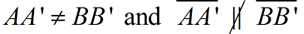.
Which transformation was applied to ΔABC ?
Choose:
 reflection translation rotation4.
In a reflection, the points of the pre-image are always the same distance away from the line of reflection as the corresponding points of the image.
Choose:
 TRUE FALSE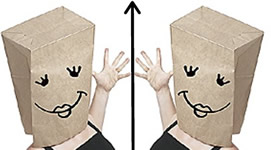5.
The diagram at the right shows a rotation centered at point P.
Find the values of x, y and w.
Choose:
 x = 134     y = 10     w = 3 x = 33.5    y = 10    w = 3 x = 33.5     y = 2.5    w = 3 x = 33    y = 2    w = 3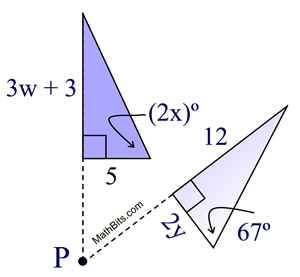6.
The drawing of a jar is created by reflecting half of the drawing over a line of reflection, m.
a) What is the width of the full drawing of the jar at its widest point?

Choose:
 16" 8" 3" 4"

b)
The jar sits on a circular base. What is the circumference of the base?

Choose:
 3π in. 6π in. 8π in. 9π in.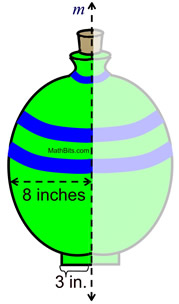7.
ΔRST is translated by the rule:
(x, y) → (x + 3, y - 4).
a) Is RS = R'S' ?
Choose:
 Yes No Sometimes

b)
If R(-2,5), what is RR' ?
Choose:
 11 8 6 58.
In a rigid transformation on ΔABC,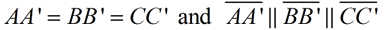. Which transformation was applied to ΔABC ?
Choose:
 reflection translation rotation9.
A rigid transformation is applied to a figure under the rule (x, y) → (-x, -y).
What effect does this transformation have on the figure?

Choose:
 90º rotation about the origin 180º rotation about the origin reflection over the y-axis reflection over the x-axis10.
In a rigid transformation on ΔABC,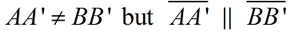.
Which transformation was applied to ΔABC ?
Choose:
 reflection translation rotation